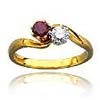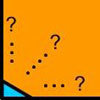# Resources tagged with: Surds

Filter by: Content type:
Age range:
Challenge level:

### There are 19 results

Broad Topics > Numbers and the Number System > Surds### Plus or Minus

##### Age 16 to 18 Challenge Level:

Make and prove a conjecture about the value of the product of the Fibonacci numbers $F_{n+1}F_{n-1}$.### Absurdity Again

##### Age 16 to 18 Challenge Level:

What is the value of the integers a and b where sqrt(8-4sqrt3) = sqrt a - sqrt b?### Irrational Arithmagons

##### Age 16 to 18 Challenge Level:

Can you work out the irrational numbers that belong in the circles to make the multiplication arithmagon correct?### Golden Eggs

##### Age 16 to 18 Challenge Level:

Find a connection between the shape of a special ellipse and an infinite string of nested square roots.### Surds

##### Age 14 to 16 Challenge Level:

Find the exact values of x, y and a satisfying the following system of equations: 1/(a+1) = a - 1 x + y = 2a x = ay### Pythagorean Fibs

##### Age 16 to 18 Challenge Level:

What have Fibonacci numbers got to do with Pythagorean triples?### Fibonacci Fashion

##### Age 16 to 18 Challenge Level:

What have Fibonacci numbers to do with solutions of the quadratic equation x^2 - x - 1 = 0 ?### In Between

##### Age 16 to 18 Challenge Level:

Can you find the solution to this algebraic inequality?### The Root of the Problem

##### Age 14 to 18 Challenge Level:

Find the sum of this series of surds.### Ab Surd Ity

##### Age 16 to 18 Challenge Level:

Find the value of sqrt(2+sqrt3)-sqrt(2-sqrt3)and then of cuberoot(2+sqrt5)+cuberoot(2-sqrt5).### Cube Roots

##### Age 16 to 18 Challenge Level:

Evaluate without a calculator: (5 sqrt2 + 7)^{1/3} - (5 sqrt2 - 7)^1/3}.### Bina-ring

##### Age 16 to 18 Challenge Level:

Investigate powers of numbers of the form (1 + sqrt 2).### Golden Mathematics

##### Age 16 to 18

A voyage of discovery through a sequence of challenges exploring properties of the Golden Ratio and Fibonacci numbers.### Strange Rectangle 2

##### Age 16 to 18 Challenge Level:

Find the exact values of some trig. ratios from this rectangle in which a cyclic quadrilateral cuts off four right angled triangles.### Fit for Photocopying

##### Age 14 to 16 Challenge Level:

Explore the relationships between different paper sizes.### Impossible Square?

##### Age 16 to 18 Challenge Level:

Can you make a square from these triangles?### Baby Circle

##### Age 16 to 18 Challenge Level:

A small circle fits between two touching circles so that all three circles touch each other and have a common tangent? What is the exact radius of the smallest circle?### Golden Construction

##### Age 16 to 18 Challenge Level:

Draw a square and an arc of a circle and construct the Golden rectangle. Find the value of the Golden Ratio.### Pentabuild

##### Age 16 to 18 Challenge Level:

Explain how to construct a regular pentagon accurately using a straight edge and compass.